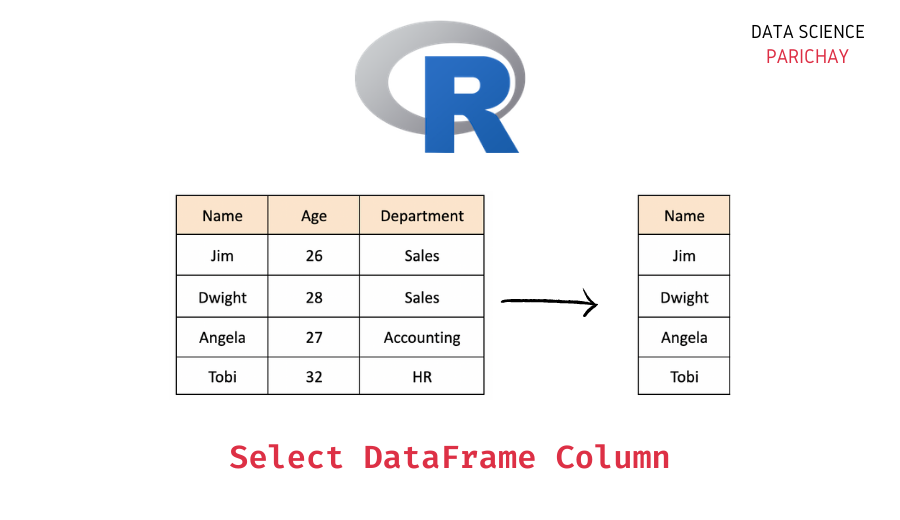# Select One or More Columns From R Dataframe

A dataframe in R is a two-dimensional data structure used to store the data in rows and columns and perform different operations on it. In this tutorial, we will look at how to select one or more columns from a dataframe in R with the help of some examples.

## How do you select columns from an R dataframe?

You can use the `select()` function available in the `dplyr` package to select one or more columns from a dataframe in R.

The following is the syntax –

`select(dataframe_input, column_names)`

Pass the dataframe as the first argument and then the column name(s) of the column(s) you want to select as comma-separated arguments.

It returns an R dataframe with the selected columns.

## Steps to select columns from dataframe in R using `select()` function

Let’s now look at a step-by-step example of selecting columns from a dataframe in R.

### Step 1 – Create a dataframe

First, we will create a dataframe that we will be using throughout this tutorial.

```# create a dataframe
employees_df = data.frame(
"Name"= c("Jim", "Dwight", "Angela", "Tobi", "Kevin"),
"Age"= c(26, 28, 29, 32, 30),
"Department"= c("Sales", "Sales", "Accounting", "HR", "Accounting")
)
# display the dataframe
print(employees_df)```

Output:

📚 Data Science Programs By Skill Level

Introductory

Intermediate ⭐⭐⭐

🔎 Find Data Science Programs 👨‍💻 111,889 already enrolled

Disclaimer: Data Science Parichay is reader supported. When you purchase a course through a link on this site, we may earn a small commission at no additional cost to you. Earned commissions help support this website and its team of writers.

```    Name Age Department
1    Jim  26      Sales
2 Dwight  28      Sales
3 Angela  29 Accounting
4   Tobi  32         HR
5  Kevin  30 Accounting```

We now have a dataframe containing information about some employees working in an office. The dataframe has columns “Name”, “Age”, and “Department”.

Let’s say you only want to select the “Name” column from the above dataframe. We’ll do that by using the `select()` function.

### Step 2 – Import the `dplyr` package

The `select()` function is defined in the `dplyr` package which has to be imported before we can actually use it.

```# import dplyr package
library("dplyr")```

Here we use the `library()` function to import the `dplyr` package.

### Step 3 – Select the column using `select()` function

Let’s now select the “Name” column from the above dataframe using the `select()` function.

```# select "Name" column
new_df = select(employees_df, "Name")
# display the dataframe
print(new_df)```

Output:

```    Name
1    Jim
2 Dwight
3 Angela
4   Tobi
5  Kevin```

We get a dataframe with only the “Name” column.

Note that you can also use the column index to select a column in the `select()` function. (Rows and columns are indexed starting from 1 in R dataframes).

```# select "Name" column using its index
new_df = select(employees_df, 1)
# display the dataframe
print(new_df)```

Output:

```    Name
1    Jim
2 Dwight
3 Angela
4   Tobi
5  Kevin```

We get the same result as above. Here, we pass column index 1 (which represents the “Name” column in the above dataframe) instead of its name to the `select()` function.

## Select multiple columns using the `select()` function

You can also use the `select()` function to select more than one column.

For example, let’s select only the “Name” and “Department” columns from the above dataframe.

```# select "Name" and "Department" columns
new_df = select(employees_df, "Name", "Department")
# display the dataframe
print(new_df)```

Output:

```    Name Department
1    Jim      Sales
2 Dwight      Sales
3 Angela Accounting
4   Tobi         HR
5  Kevin Accounting```

The resulting dataframe contains only the “Name” and “Department” columns.

You might also be interested in –

••